Sunil Ray — Published On May 17, 2015 and Last Modified On July 19th, 2020

### Introduction

The beauty of art lies in the message it conveys. At times, reality is not what we see or perceive. The endless efforts from the likes of Vinci and Picasso have tried to bring people closer to the reality using their exceptional artworks on a certain topic/matter.

Data scientists are no less than artists. They make paintings in form of digital visualization (of data) with a motive of manifesting the hidden patterns / insights in it. It is even more interesting to know that, the tendency of human perception, cognition and communication increases when he / she gets exposed to visualized form of any content/data.

There are multiple tools for performing visualization in data science. In this article, I have demonstrated various visualization charts using Python.

### What does it take to make visualization in Python?

Not much ! Python has already made it easy for you – with two exclusive libraries for visualization, commonly known as matplotlib and seaborn. Heard of them?

Matplotlib: Python based plotting library offers matplotlib with a complete 2D support along with limited 3D graphic support. It is useful in producing publication quality figures in interactive environment across platforms. It can also be used for animations as well. To know more about this library, check this link.

Seaborn: Seaborn is a library for creating informative and attractive statistical graphics in python. This library is based on matplotlib. Seaborn offers various features such as built in themes, color palettes, functions and tools to visualize univariate, bivariate, linear regression, matrices of data, statistical time series etc which lets us to build complex visualizations. To know more about this library, check this link.

### What are the different visualizations I can make?

Last week, A comprehensive guide on Data Visualization was published to introduce you to the most commonly used visualizations techniques. We recommend you to refer that before proceeding further, in case you haven’t.

Below are the python codes with their output. I have used following data set to create these visualization: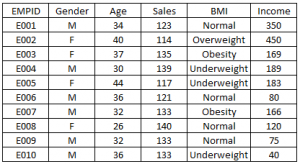#### Import Data Set:

```import matplotlib.pyplot as plt
import pandas as pd

```

#### Histogram :

```fig=plt.figure() #Plots in matplotlib reside within a figure object, use plt.figure to create new figure
#Create one or more subplots using add_subplot, because you can't create blank figure
#Variable
ax.hist(df['Age'],bins = 7) # Here you can play with number of bins
Labels and Tit
plt.title('Age distribution')
plt.xlabel('Age')
plt.ylabel('#Employee')
plt.show()```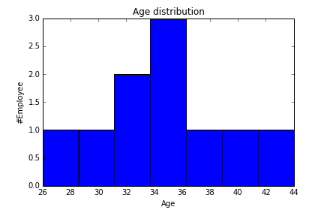#### Box Plot

```import matplotlib.pyplot as plt
import pandas as pd
fig=plt.figure()
#Variable
ax.boxplot(df['Age'])
plt.show()```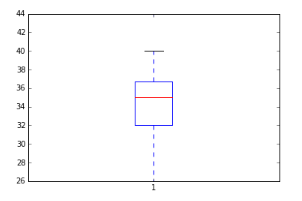#### Violin Plot

```import seaborn as sns
sns.violinplot(df['Age'], df['Gender']) #Variable Plot
sns.despine()```

#### Bar Chart

```var = df.groupby('Gender').Sales.sum() #grouped sum of sales at Gender level
fig = plt.figure()
ax1.set_xlabel('Gender')
ax1.set_ylabel('Sum of Sales')
ax1.set_title("Gender wise Sum of Sales")
var.plot(kind='bar')```#### Line Chart

```var = df.groupby('BMI').Sales.sum()
fig = plt.figure()
ax1.set_xlabel('BMI')
ax1.set_ylabel('Sum of Sales')
ax1.set_title("BMI wise Sum of Sales")
var.plot(kind='line')```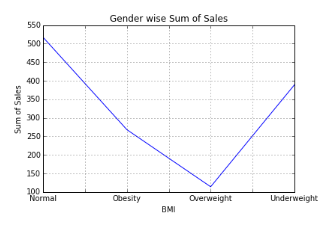#### Stacked Column Chart

```var = df.groupby(['BMI','Gender']).Sales.sum()
var.unstack().plot(kind='bar',stacked=True,  color=['red','blue'], grid=False)```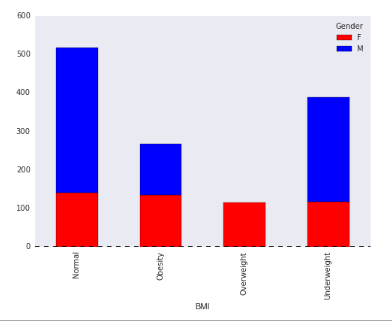Dataframe.unstack() returns a Data Frame having a new level of column labels whose inner-most level consists of the pivoted index labels. Read more about dataframe.unstack here.

#### Scatter Plot

```fig = plt.figure()
ax.scatter(df['Age'],df['Sales']) #You can also add more variables here to represent color and size.
plt.show()```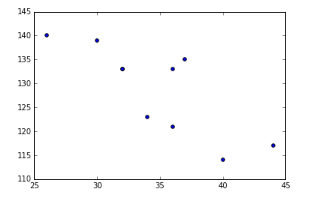#### Bubble Plot

```fig = plt.figure()
ax.scatter(df['Age'],df['Sales'], s=df['Income']) # Added third variable income as size of the bubble
plt.show()```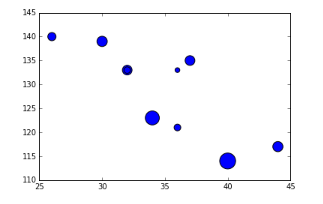#### Pie chart

```var=df.groupby(['Gender']).sum().stack()
temp=var.unstack()
type(temp)
x_list = temp['Sales']
label_list = temp.index
pyplot.axis("equal") #The pie chart is oval by default. To make it a circle use pyplot.axis("equal")
#To show the percentage of each pie slice, pass an output format to the `autopct`parameter plt.pie(x_list,labels=label_list,autopct="%1.1f%%") plt.title("Pastafarianism expenses") plt.show()```#### Heat Map

```import numpy as np
#Generate a random number, you can refer your data values also
data = np.random.rand(4,2)
rows = list('1234') #rows categories
columns = list('MF') #column categories
fig,ax=plt.subplots()
ax.pcolor(data,cmap=plt.cm.Reds,edgecolors='k')
ax.set_xticks(np.arange(0,2)+0.5)
ax.set_yticks(np.arange(0,4)+0.5)
# Here we position the tick labels for x and y axis
ax.xaxis.tick_bottom()
ax.yaxis.tick_left()
#Values against each labels
ax.set_xticklabels(columns,minor=False,fontsize=20)
ax.set_yticklabels(rows,minor=False,fontsize=20)
plt.show()```
```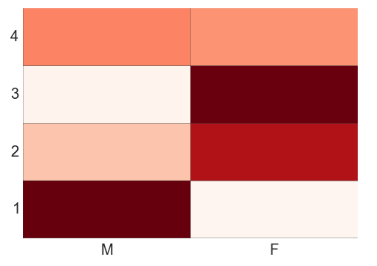```

You can attempt to plot a heat based on two variables like Gender on x-axis, BMI on Y-axis and Sales values as data points. Please share your code and output in comment section.

### End Notes

By now, you must have realized, how beautifully data can be presented using visualization. I find performing visualization in Python much easier as compared to R. In this article, we discussed about deriving various visualizations in Python. In this process, we made use of matplotlib and seaborn in python.  In the subsequent articles we will explore map visualization and word cloud in python.

Do you have any questions on visualization methods / techniques / selection? Feel free to discuss it with us.

### If you like what you just read & want to continue your analytics learning, subscribe to our emails, follow us on twitter or like our facebook page.###### Sunil Ray

I am a Business Analytics and Intelligence professional with deep experience in the Indian Insurance industry. I have worked for various multi-national Insurance companies in last 7 years.

### Our Top Authors

•